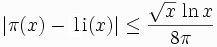# 2657

This number is a prime.The American mathematician Lowell Schoenfeld (1920-2002) proved that if the Riemann hypothesis was true, thenfor all x greater than or equal to 2657.2657# + 1 is the smallest titanic primorial prime of form p# + 1. [Loungrides]2657 has a peculiar property. It can be written as the sum of a power of its first digit and a power of its last digit, i.e., 2657 = 2^8 + 7^4. [Leonardis]